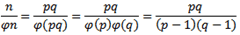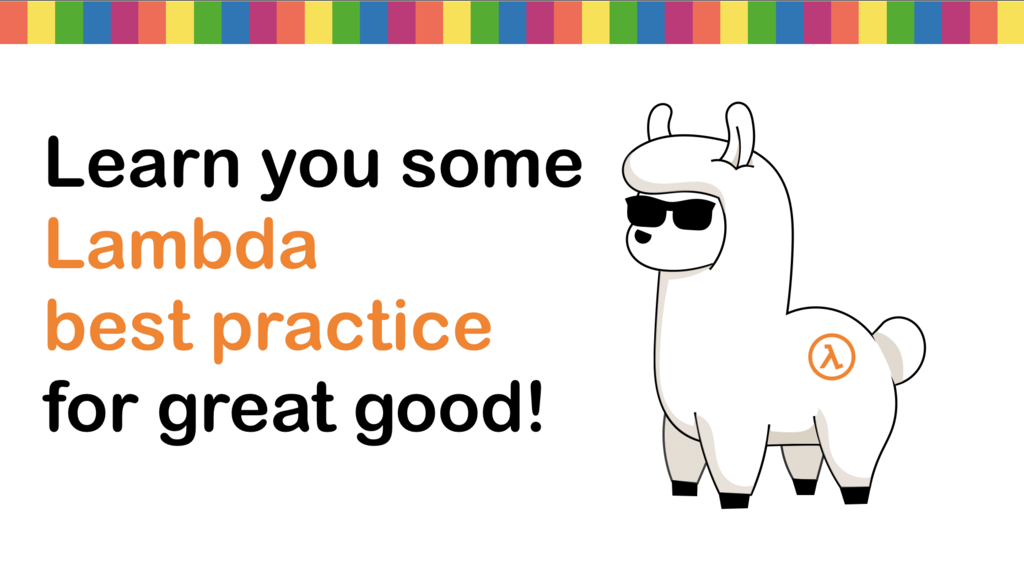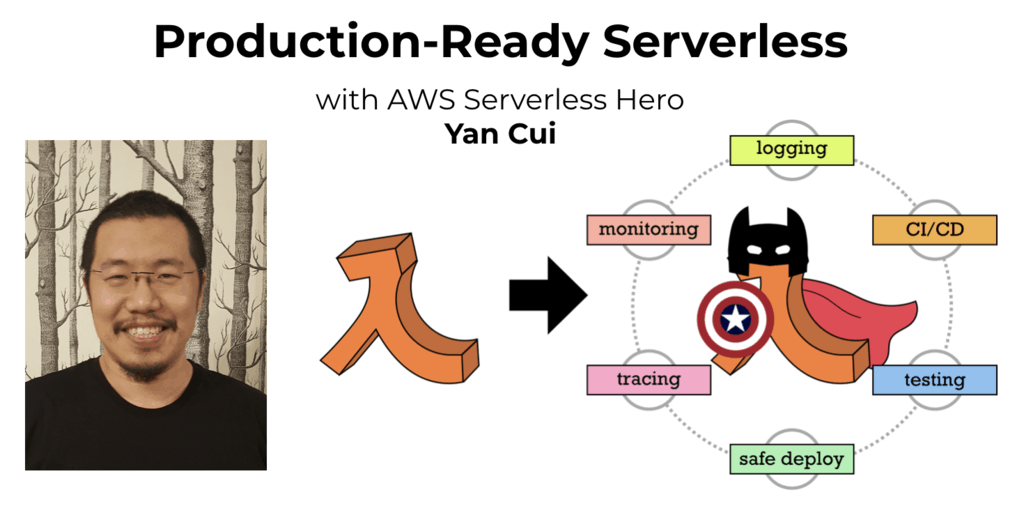# Project Euler – Problem 70 Solution

Check out my new course Learn you some Lambda best practice for great good! and learn the best practices for performance, cost, security, resilience, observability and scalability.

#### Problem

Euler’s Totient function, ?(n) [sometimes called the phi function], is used to determine the number of positive numbers less than or equal to n which are relatively prime to n. For example, as 1, 2, 4, 5, 7, and 8, are all less than nine and relatively prime to nine, ?(9)=6.

The number 1 is considered to be relatively prime to every positive number, so ?(1)=1.

Interestingly, ?(87109)=79180, and it can be seen that 87109 is a permutation of 79180.

Find the value of n, 1 < n < 107, for which ?(n) is a permutation of n and the ratio n/?(n) produces a minimum.

#### Solution

```// generate all prime numbers under <= this max
let max = 10000I

// initialise the list with 2 which is the only even number in the sequence

// only check the prime numbers which are <= the square root of the number n
let hasDivisor n =
|> Seq.takeWhile (fun n' -> n' <= bigint(sqrt(double(n))))
|> Seq.exists (fun n' -> n % n' = 0I)

// only check odd numbers <= max
let potentialPrimes = Seq.unfold (fun n -> if n > max then None else Some(n, n+2I)) 3I

// populate the prime numbers list
for n in potentialPrimes do if not(hasDivisor n) then primeNumbers <- primeNumbers @ [n]

// use the same hasDivisor function instead of the prime numbers list as it offers
// far greater coverage as the number n is square rooted so this function can
// provide a valid test up to max*max
let isPrime n = if n = 1I then false else not(hasDivisor(n))

// define function to find the prime denominators for a number n
let getPrimeFactors n =
let rec getPrimeFactorsRec denominators n =
if n = 1I then denominators
else
let denominator = primeNumbers |> Seq.filter (fun x -> n % x = 0I) |> Seq.head
getPrimeFactorsRec (denominators @ [denominator]) (n/denominator)
getPrimeFactorsRec [] n

// define Euler's totient function
let totient n =
if n = 1I then 1I
else if isPrime n then n-1I
else
let primeFactors = getPrimeFactors n |> Seq.distinct
n * (primeFactors |> Seq.map (fun n' -> n'-1I) |> Seq.reduce (*)) / (primeFactors |> Seq.reduce (*))

// define function to check if two numbers are permutations of each other
let isPermutation a b =
let aArray = a.ToString().ToCharArray() |> Array.sort
let bArray = b.ToString().ToCharArray() |> Array.sort
if Array.length aArray <> Array.length bArray then false
else Array.forall2 (fun aChar bChar -> aChar = bChar) aArray bArray

// check semi-primes less than 10 million
|> Seq.collect (fun n ->
|> Seq.filter (fun n' -> n' > n)
|> Seq.map (fun n' -> n * n'))
|> Seq.filter (fun n' -> n' > 8000000I && n' < 10000000I)
|> Seq.map (fun n -> (n, totient n))
|> Seq.filter (fun (n, n') -> isPermutation n n')
|> Seq.minBy (fun (n, n') -> double(n) / double(n'))
```

According to MathWorld the totient function of n equals n – 1if n is a prime, under normal circumstances n divided by totient n is minimal if n is a prime, but then they wouldn’t be permutations of each other as n cannot be the permutation of n – 1.

A semi-prime on the other hand, n, where p and q are primes and n = pq, its totient function equals (p – 1)(q – 1), and therefore:and is most likely the source of the answer.

The solution here generates all the semi-primes under 10 million and looks for the semi-prime n which returns the smallest n/totient n. The larger n is the smaller n/totient n is, hence to reduce the amount of computation required I only check for numbers greater than 8 million.Hi, I’m Yan. I’m an AWS Serverless Hero and the author of Production-Ready Serverless.

I specialise in rapidly transitioning teams to serverless and building production-ready services on AWS.

Are you struggling with serverless or need guidance on best practices? Do you want someone to review your architecture and help you avoid costly mistakes down the line? Whatever the case, I’m here to help.Check out my new course, Learn you some Lambda best practice for great good! In this course, you will learn best practices for working with AWS Lambda in terms of performance, cost, security, scalability, resilience and observability. Enrol now and enjoy a special preorder price of £9.99 (~\$13).Are you working with Serverless and looking for expert training to level-up your skills? Or are you looking for a solid foundation to start from? Look no further, register for my Production-Ready Serverless workshop to learn how to build production-grade Serverless applications!